CSE4100 Java Programming Paper Editing Services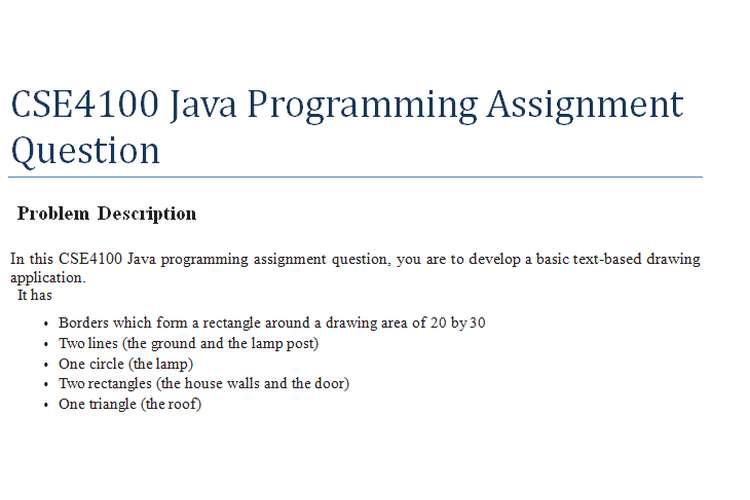Problem Description

In this CSE4100 Java programming assignment, you are to develop a basic text-based drawing application.
It has

• Borders which form a rectangle around a drawing area of 20 by 30
• Two lines (the ground and the lamp post)
• One circle (the lamp)
• Two rectangles (the house walls and the door)
• One triangle (the roof)
• A string of text (“FOR SALE” displayed vertically)

In Part 2, you will develop a menu program that allows the user to progressively create a drawing (by adding shapes and removing shapes), save the definition of the drawing in a text file and read it back to reconstruct it.

In Part 1, your main task is to develop the classes whose instances are objects that make up a drawing. Essentially, they are classes that represent windows and shapes.

Windows

Each drawing is a window on which shapes can be drawn. A window has, among other things,

• the height
• the width
• the border character that is used to draw the borders

The window’s height and width represent the drawing area, excludingthe borders. That is, a window of height 20 and width 30 has 20 x 30 = 600 cells each of which contains a blank or a non-blank character.
There are five kinds of shapes that your programs can draw:

• Lines
• Rectangles
• Triangles
• Circles
• Texts (regarded as a kind of shape) Each shape has
• a base point (that will be further explained as we describe the specific shapes), and
• a display character i.e. the character that is used to display the shape

Note about extension for Part 2:In Part 2, we will add two attributes to a shape: id and description. The id allows us to identity the shape in a drawing. The description allows us to add some information, for example what the shape represents in a drawing, e.g a rectangle   can represent a door of a house.

Lines

Consider, as an example, the line below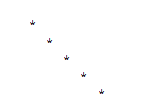Suppose the top point of the line is at position 10, 15. And suppose we take it to be the base point of the line. Then the line can be specified as follows:

• The row position of its base point, which is 10
• The column position of its base point, which is 15
• Its length, which is 4
• Its row increment is 1
• Its column increment is 1
• And its drawing character, which is ‘*’

The row increment 1 and column 1 signifies that the line goes down and goes to the right from the base point. For our drawing board, row increment and column increment can only take values -1, 0, or 1. In  other words, a line can be horizontal, vertical or can incline at an angle of 45 degrees.

Consider, as an example, the rectangle below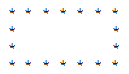Suppose the top-left point is at position 10, 15.  This point will be taken to be the base point.  The line can be specified as follows:

• The row position of its base point, which is 10
• The column position of its base point, which is 15
• Its height, which is 3
• Its width is 6
• And its drawing character, which is ‘*’

Triangles

Our drawing board will draw only isosceles triangle. Consider, as an example, the triangle below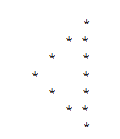Suppose the left-most point is at position 10, 15. This point, where the two equal sides meet, will be  referred to as the base pointof the triangle. The directed perpendicular line segment from the base point    to the opposite side is referred to as the height vectorof the triangle. The height vector can have one of   four possible directions. It can go:

1. Right(as in the above example). We specify this direction by taking its row increment to be 0 and column increment to be 1.
2. Left, with row increment 0 and column increment -1.
3. Up, with row increment -1 and column increment 0.
4. Down, with row increment 1 and column increment 0.

In addition, the length of the side opposite the base point is twice the length of the height vector. The triangle can be specified as follows:

• The row position of its base point, which is 10
• The column position of its base point, which is 15
• Its height, the length of the height vector, which is 3
• Its row increment of the height vector, which is 0
• Its column increment of the height vector, which is 1
• And its drawing character, which is ‘*’

Circle

Consider the circle displayed below, which is a circle of radius 2.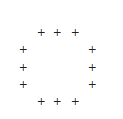The base point of a circle is its center. A circle is specified by

• The row position of its base point (its center)
• The column position of its base point (its center)
• And its drawing character, which is ‘+’

For our drawing boards,  we draw a circle by plotting 20 points on its circumference.   When a circle is of small size, some of the 20 points overlap. Obviously, the display is often only a rather crude approximation of a circle. (Hint: In reference to a coordinate system whose origin is the center Oof the circle, a point on the circle has coordinates = R cos(α) and = R sin(α), where Ris the radius    and α is the angle between the x-axis to OM).

Consider, as an example, the text display below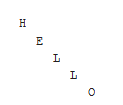Suppose letter ‘H’ is at position 10, 15. This is the base point. This “text shape” can be specified as  follows:

• The row position of its base point, which is 10
• The column position of its base point, which is 15
• Its text itself, which is “HELLO”
• Its row increment is 1
• Its column increment is 1

The row increment 1 and column 1 signifies that the line goes down and goes to the right from the base point. For our drawing board, as for the lines, row increment and column increment of a text shape can  take values -1, 0, or 1.

The Window class must have

• The constructor

Window(int numberOfRows, int numberOfColumns, char borderCharacter)

• The method to add a shape

• The method to remove a shape

void removeShape(String id)

You are not required to implement this method for Part 1.

• The method to display the drawing on the screen

void display()

Row value increases from top to bottom, and column value increases from left to right. The top-left cell  has row = 1 and column = 1.

The Line class must have the constructor

Line(int rowBase, int colBase, int length, int rowIncrement, int colIncrement, char drawingCharacter)

The Rectangle class must have the constructor

Rectangle(int rowBase, int colBase, int height, int width, char drawingCharacter)

The Triangle class must have the constructor

Triangle(int rowBase, int colBase, int height, int rowIncrement, int colIncrement, char drawingCharacter)

The Circle class must have the constructor

Circle(int rowBase, int colBase, int radius, char drawingCharacter)

The Text class must have the constructor

Text(int rowBase, int colBase, String text, int rowIncrement, int colIncrement)

In addition, every shape class (Line, Rectangle, Triangle, Circle, Text) must also have the method to       draw itself on a Window

void draw(Window window)

1. Design and implement

• The class to represent a drawing window
• The abstract class Shape
• The class that represents a line

2. Test your classes with the Eight Lines program below. The program must be able to run with your classes without any change.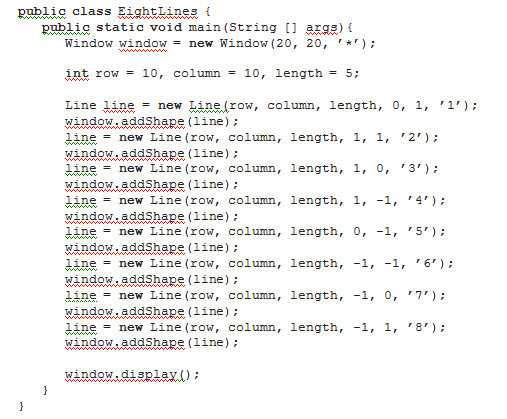The program should produce the output: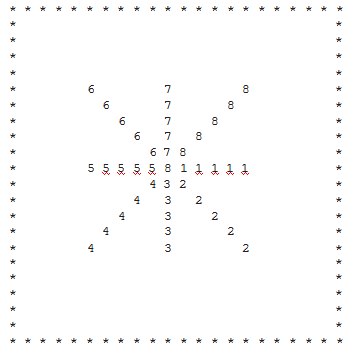1. Design and implement the rest of the shapes
2. Test your classes with the program HouseForSale below. It should produce the drawing on page 2.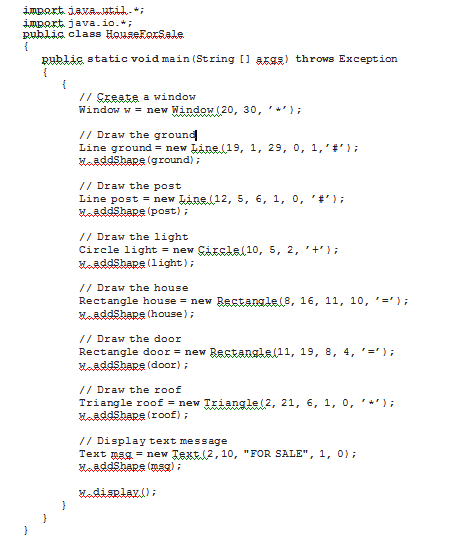It is required that the classes you develop must allow the HouseForSale program to run without any changes.

• Submit all the Java files that you have developed in the tasks above.
• The code has to run under Unix on the latcs8 machine.
• You submit your files from your latcs8 account.  Make sure you are in the same directory as the    files you are submitting.
• Submit each file separately using the submit command. For example, for a file called (say) Win- dow.java:

submit IOO Window.java

• After submitting the files, you can run the following command that lists the files submitted from   your account:

verify

• You can submit the same filename as many times as you like before the assignment deadline; the previously submitted copy will be replaced by the latest one.

Marking Scheme Overview

Part 1 of the assignment has the total of 100 marks, which are distributed as follows:

• Implementation (Execution of code) 90 marks (Do all parts of the programs execute correctly?    Note your programs must compile and run to carry out this implementation marking.)
• Code Design, Layout and Documentation 10 marks (Does the program conform to specifications? Does the program solve the problem in a well-designed manner? Does the program follow good programming practices? Does the indentation and code layout follow a good, consistent standard? Are the identifiers meaningful? Are comments useful in explain what and how the program works? (Javadoc comments are optional.)